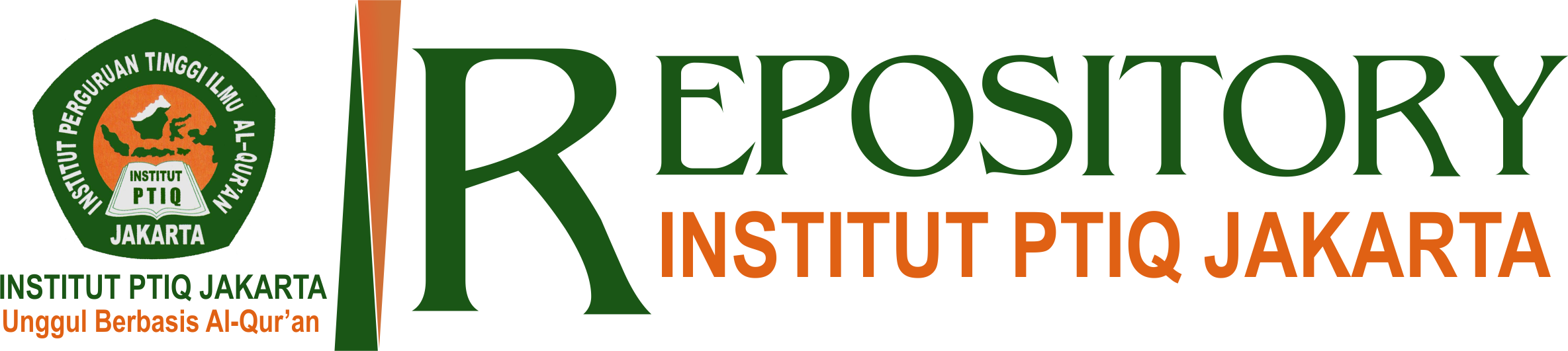#### Pengaruh Motivasi Belajar dan Profesionalisme Guru Terhadap Prestasi Siswa

Sarnoto, Ahmad Zain (2017) Pengaruh Motivasi Belajar dan Profesionalisme Guru Terhadap Prestasi Siswa. PROFESI: Jurnal Ilmu Pendidikan dan Keguruan, 6 (2). ISSN 2797-0701

## Abstract

In this reaserch, the writer uses survey method with correlation approach towards quantitative data wich obtained from object of reaserch which are Muhammadiyah high school of 7, sawangan depok, jawa barat, with the study sample of 115 student’s of Muhammadiyah high school of 7, sawangan depok, jawa barat, at the first semester of the academic year 2016-2017. In this reaserch writer were uses questionnaires, observation, and documentation. For data analyse , writer use data analyse technique with the calculation method of computerized system (SPSS) for windows 22 version with correlation and regression analyse type are descriptively elaborated. The reaserch shows that.

First, from computeraized count (SPSS) there is a significant positive influence between studies motivation towards value PAI students show that for value t-cuont studies motivation variabel (X1) is 2,50< value t-table 1,95 ( n-k = 115-2=113) and significant value is 0,011< a = 0,05 because value t-cuont studies motivation variable (X1) is 2,580 > value t-table = 1,95 and significant value 0,011 < a = 0,05 so HO reviews and H1 ricive. This is mean studies motivation variable (X1) influence significantly and positive to variable value learn PAI (Y).

Second, value t-cuont teacher’s professionalism variabel (X2) is 7,456> value t-table 1,95 ( n-k = 115-2=113) and significant value is 0,000 < a = 0,05 because value t-cuont teacher’s professionalism variabel variable (X2) is 7,456 > value t-table = 1,95 and significant value 0,000< a = 0,05 so Ho reviews and H1 ricive. This is mean studies motivation variable (X1) influence significantly and positive to variable value learn PAI (Y).

Third, from table summary type there is coefficient determination (R2) independent variable and dependent variable is 0,580 (58%), that mean the amount of contribution independent variabel towards dependent variable is 58% and the rest is 42% (100%-58% = 42%) from variable or from onather factor that not search yet in this searching. from table summary type rich strength value relation (R) between studies motivation variable (X1) and teacher’s professionalism variabel (X2) to learn value PAI (Y) is 0,726 (76,2%). That show the relation between studies motivation variable (X1) and teacher’s professionalism variabel (X2) to learn value PAI (Y) is strong because at the range 0,600 – 0,799

Item Type: Article 300. Ilmu Sosial > 370. Pendidikan Jurnal Online Institut PTIQ Jakarta Andi Jumardi 04 Dec 2022 05:53 04 Dec 2022 05:53 https://repository.ptiq.ac.id/id/eprint/858